# Price List

## Primary Math International

### KindergartenLesson Book (Kindergarten)

The Lesson Book is provided to each student, and functions as a textbook and a notebook.

\$20.00 USDWorkbook (Kindergarten)

The Workbook provides additional math problems based on the contents of the Lesson Book.

\$16.00 USD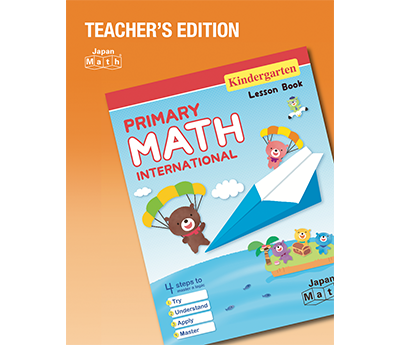Teacher’s Edition (Kindergarten)

The Teacher's Edition describes ideas behind the classroom activities and points of instruction to assist teachers.

\$120.00 USD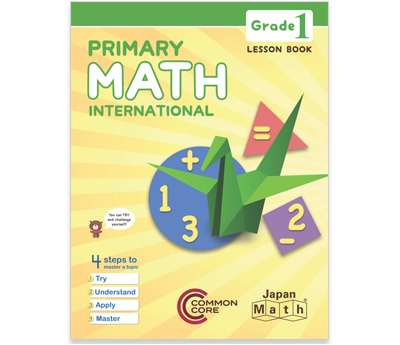Lesson Book (Grade 1)

The Lesson Book is provided to each student, and functions as a textbook and a notebook.

\$20.00 USD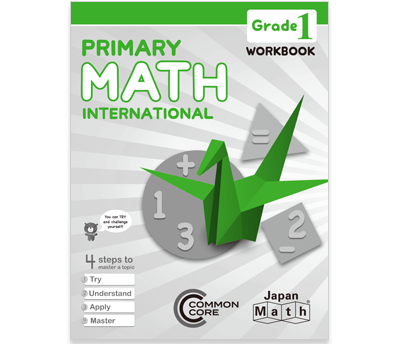The Workbook provides additional math problems based on the contents of the Lesson Book.

\$16.00 USD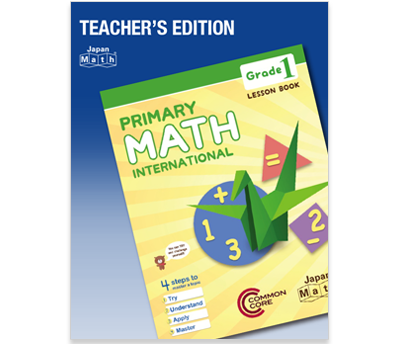Teacher's Edition (Grade 1)

The Teacher's Edition describes ideas behind the classroom activities and points of instruction to assist teachers.

\$120.00 USD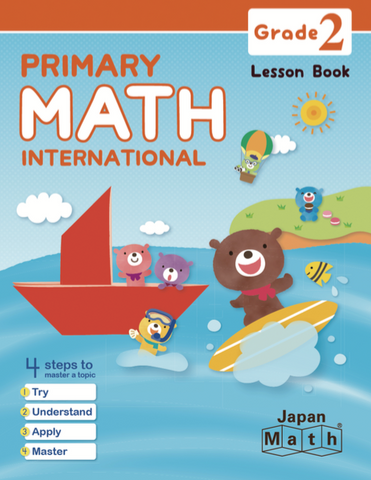Lesson Book (Grade 2)

The Lesson Book is provided to each student, and functions as a textbook and a notebook.

\$23.50 USD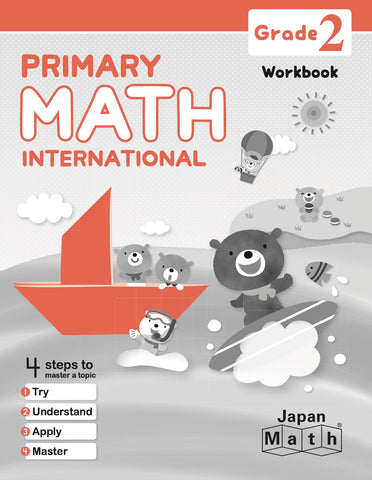The Workbook provides additional math problems based on the contents of the Lesson Book.

\$16.00 USDTeacher's Edition (Grade 2)

The Teacher's Edition describes ideas behind the classroom activities and points of instruction to assist teachers.

\$120.00 USD

## ManipulativeJapan Math Block Set (Kindergarten - Grade 1)

A set of essential tools for young children designed to help them understand the concept of numbers.

\$18.00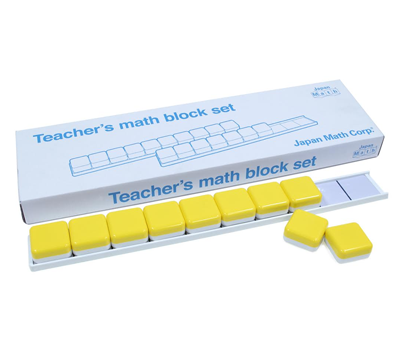Teacher's Block Set (Kindergarten - Grade 1)

Big magnetic math blocks to be used on whiteboards for explaining the operation of blocks to students.

\$98.00